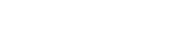Price \$27.24
Enroll Now

#### Approx 2 Hours To Complete Learn Basics Concept Of Trigonometry Functions And Identities in English Language

Introduction to Trigonometry
Trigonometric ratios of an acute angle of a right-angled triangle. Proof of their existence (well defined). Values of the trigonometric ratios of 30, 45 and 60 Degrees. Relationships between the ratios.

Trigonometric Identities
Proof and applications of the identity sin2A + cos2A = 1. Only simple identities to be given

#### Requirements

8 th to 10th Grade students

#### Syllabus

Introduction to Trigonometry

Trigonometric ratios of an acute angle of a right-angled triangle. Proof of their existence (well defined). Values of the trigonometric ratios of 300, 450 and 600. Relationships between the ratios.

Trigonometric Identities

Proof and applications of the identity sin2A + cos2A = 1. Only simple identities to be given

#### What you will learn

• Trigonometry Ratios

• Trigonometry Ratios And Identities In Detail• 0 Instructor Rating
• 0 Reviews
• 0 Students
• 1 Courses
• #### AkashA

Qualification : Btech Mechanical

• Stars 5
• 0%

• Stars 4
• 0%

• Stars 3
• 0%

• Stars 2
• 0%

• Stars 1
• 0%

## 0

Course Rating

Price \$27.24
Enroll Now

##### Includes
Do You have a Question